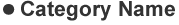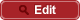• HOME
• RIKEN-QHP
• Observation of $\Xi_c (2930)^0$ and updated measurement of $B^- \to K^- \Lambda_c^+ \bar{\Lambda}_c^-$

##PrePrint No. RIKEN-QHP-355 Y. B. Li et al., (including M. Takizawa) (Belle Collaboration) Observation of $\Xi_c (2930)^0$ and updated measurement of $B^- \to K^- \Lambda_c^+ \bar{\Lambda}_c^-$ arXiv: 1712.03612  (org) 2017.12